## cNORM - Examples

In the following we would like to present a few more examples of the use of cNORM. You can retrace some of these with the data contained in the package. To do this, first install cNORM and activate the library.

### Content

First, let us return to the example of modeling reading comprehension already presented on the previous pages. The supplied 'elfe' data set contains only a grouping variable 'group' and a raw score variable 'raw'. In this example, the grouping variable also represents the continuous explanatory variable, since the standardization only took place at the beginning and middle of the school year. The following syntax summarizes the modeling process:

# The 'elfe' data set is already included.
# Calculation of the manifest percentiles and subsequent
# normal rank transformation to determine the location
# and modeling of the regression model

model.elfe <- cnorm(raw=elfe\$raw, group=elfe\$group)

# Numerical check of the bijectivity between raw score and normal score

checkConsistency(model.elfe)

# Illustration of R2 by number of predictors

plot(model.elfe, "subset", type=0)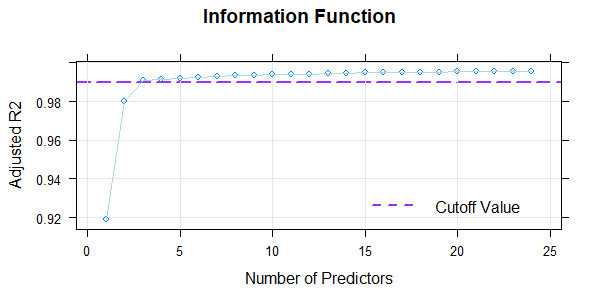# Illustration of percentile curves of the identified best model

plot(model.elfe, "percentiles")

# Alternatively, a whole series of charts can be generated, whereby
# the number of predictors is incremented by one at a time.
# If no further information is given, the chart is set to actually
# occurring raw scores and age groups of the original data set.

plot(model.elfe, "series")

In this case, R2 already exceeds 99% with only three predictors (plus intercept) in the regression function. The requirement of biuniqueness is fulfilled within the entire age range. Modeling in this example is therefore very simple and unproblematic.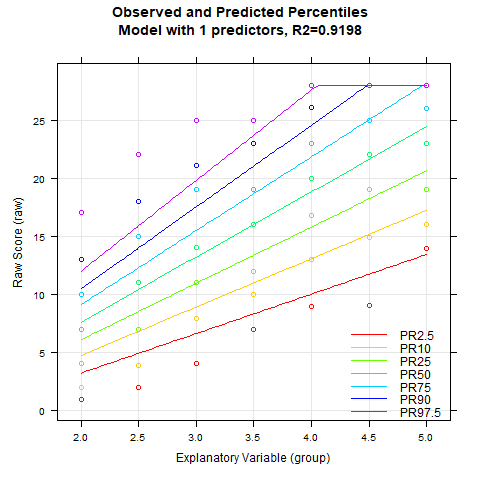The animated chart shows how the modelled percentile curves approximate the manifest data better and better with an increasing number of predictors. On the one hand, R2 increases with each additional predictor, on the other hand, you can also see unplausible waves occuring in the upper and lower percentile curves when there are too many predictors. These waves indicate a model overfit. Therefore, regression equations with more than five predictors should not be selected for this data set. The models with three, four or five predictors differ only minimally in terms of R2 and represent similarly good approximations of the manifest data.
Please note that applying the model to age ranges or locations not included in the manifest data should always be done with caution. The plausibility of the percentile curves should always be checked meticulously in these cases!

Finally, norm tables can be generated. You can choose whether to transform a series of raw scores into normal scores or vice versa.

# Transforms a specified series of normal scores into raw scores for
# third graders, ninth month of school year (explanatory variable = 3.75)

normTable(3.75, model.elfe, step=1, minNorm=25, maxNorm=75)

# Alternative: Output of T-scores for a series of raw scores

rawTable(3.75, model.elfe, step=1, minNorm=25, maxNorm=75, minRaw=0, maxRaw=28)

Output:
--------------

 raw norm 1 0 25.0 2 1 25.0 3 2 25.0 4 3 25.0 5 4 25.2 6 5 26.8 7 6 28.5 8 7 30.2 9 8 31.9 10 9 33.6 ... ... ...

### 2. Modeling of vocabulary across age 2 to 17

Next, the use of a continuous age variable will be demonstrated. For this purpose, the package contains an unselected sample taken form the standardization of a vocabulary test (data set: 'ppvt'). For the calculation of the percentiles we use a 'Sliding Window' this time. In addition, instead of the discrete grouping variable we use the continuous age variable as explanatory variable:

# Use of the data set 'ppvt' and calculation of the manifest percentiles
# and normal scores via 'sliding window'. The size of the window is 1.0
# years. The function adds information on sample size and distribution
# parameters to each case.

model.ppvt <- cnorm(raw=ppvt\$raw, age=ppvt\$age, width=1)

# The Output shows a high R2 > 0.99 with 5 terms.
# Illustration of R2 and information criterion Mallow's Cp

plot(model, "subset", type=1)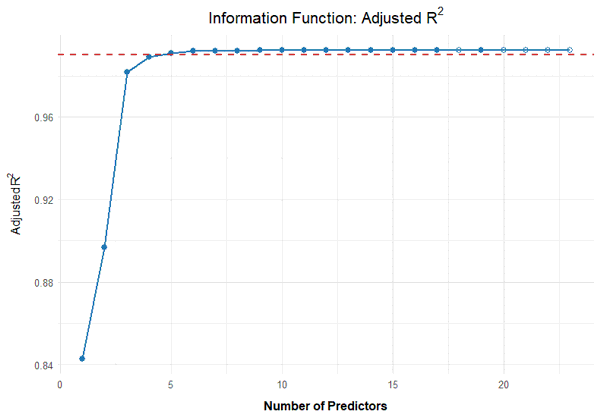# Checking the limits of model validity via first order derivative
# to location outside the age range of the test (= horizontal interpolation)
# The gradient should not fall below zero.

plot(model.ppvt, "derivative", minAge=2, maxAge=20, minNorm=20, maxNorm=80)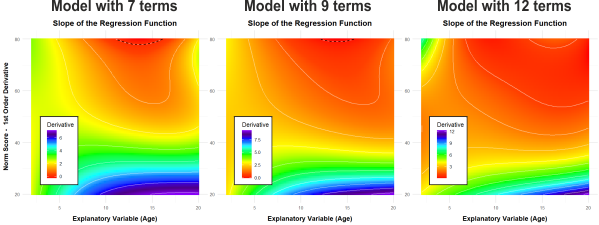In fact, the original data set does not include subjects over 17 years of age. However, in order to test the model validity beyond the age group examined, the age range in the chart was extended to 20. Orange or red zones indicate very low gradients and therefore a ceiling effect. However, violations of bijectivity or implausible curves seem to occur only outside the actual age range and/or in very high performance ranges. Nevertheless, the model validity is also checked numerically:

# Numerical check of model validity and inconsistent percentile curves

checkConsistency(model.ppvt)

# Result: Gradient above zero throughout the actual age range.

# All further steps are performed in the same way as in example 1

plot(model.ppvt, "percentiles")
normTable(10.2, model.ppvt, step=1, minNorm=25, maxNorm=75) # Age 10.2

The main difference between the first and the second example is that a continuous age variable was used. The manifest percentiles were determined continuously (rankBySlidingWindow) rather than using a grouping variable. Accordingly, the modeling must also be carried out with the continuous age variable.In this example a good fit is obtained very quickly. If - as in the default setting - the age course is set to a curvilinear trend (t = 3) and the power of the location is set to k=5, then a high fit can already be achieved with only 5 predictors. In the model with two predictors, overlapping percentile curves occur, which is a violation of bijectivity. Such a model must therefore be rejected in any case. R2 exceeds 99% with only four predictors. If the number of predictors is above seven, the lowest percentile curve starts to change its curvature in the upper age range. This change is implausible, since all other percentiles are curved to the right without exception. Overall, it can therefore be stated that the regression equations with four to seven predictors all represent plausible approximations to the manifest data with high model fit.

### 3. Biometry: Modeling of the BMI across age 2 to 25

Not only psychological variables but also biometric data can be modeled using cNORM. For this purpose, a data set of the Center of Disease Control (CDC, 2012) is used, which is supplied in the package (description can be retrieved using ?CDC) and which contains raw data about the development of the body mass index over age. Since this is a large data set, the ranking is carried out using a grouping variable to reduce the calculation effort. All further modeling steps are carried out with the continuous variables:

# Determining the model for the BMI variable

model.bmi <- cnorm(group=CDC\$group, raw=CDC\$bmi)

# The animated gif illustrated below was generated with the
# 'plotPercentileSeries' function

plot(model.bmi, "series", start=1, filename="bmichart")

With the plot(model, "series") function, model fit and model validity for an increasing number of predictors can be checked very easily. The figure caption includes the according R2 of each model:The example also shows that a high model fit can usually be achieved very quickly and easily. With 10 predictors or more, the fitted percentile curves are largely stable. R2 amounts to 99.4 % then. The BMI data show a more complex age progression. In such cases, it may help to increase the parameter t, e.g. to t = 4.

### 4. Processing time: Modeling of reading fluency across grades 2 to 5

Reaction or processing times are usually extremely skewed to the right, since there is a lower, but often no or only a very generous upper limit of the processing time. Limits of the measuring equipment or floor or ceiling effects due to automatization can further contribute to skewness. The modeling of processing times is demonstrated here with the subtest word recognition speed of the ELFE-II reading comprehension test (data not included in cNORM for copyright reasons). In this subtest, words are presented for a limited time only. For each word, the participants must decide whether it represented an animal, a plant or an artificial object. Based on the correctness of the answers, the presentation time of the words is changed stepwise until a threshold is reached at which the words can just barely be read correctly. The lower limit of the presentation time is 150 ms.Visual inspection of the boxplots per grade reveals that most children above grade three manage to correctly identify the words within a presentation time of only 150 ms. This means that above grade three, the subtest no longer discriminates adequately between proficient and poor readers. Even in the third grade a good discrimination is only achieved for poor performances. This effect can be explained by the fact that decoding is automatized in most students during the first three to four school years. (Please note that ELFE-II ist a German reading comprehension test. The results do not necessarily hold true for other languages, especially for deep orthographies.) However, reading fluency does not only develop extremely fast during elementary school, but the development also shows a wide interindividual spread, which further contributes to the skewness of the distributions. Since the main aim of the subtest is to identify children with particularly low performance, we subsequently focus on the poorest processing times. (Note that in this case high percentiles reflect high processing times, i.e. poor performance!

# Data preparation and modeling
# (elfe2 dataset not included

# Output of model fit and series of percentile charts

print(model.speed)
plot(model.speed, "series", percentiles = c(.8, .9, .95))

Even with 24 predictors, R2 does not reach .99. Moreover, increasing the number of predictors above 8 does only marginally improve R2. A large number of predictors could therefore lead to a model overfit. The preliminarily specified criterion of R2 = .99 must therefore be reduced. The visual inspection of the percentile curves speaks for a solution with only four predictors, even if in this case R2 is only at .935: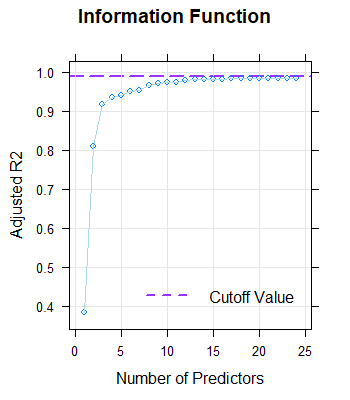10.384571185920.38338165-437.45
20.809176849020.80911178-1522.13
30.91876285310.9184298-2298.09
40.93560065920.9353189-2513.80
50.94055017030.9402846-2588.71
60.95045767030.9502215-2753.25
70.95542076490.9551964-2824.69
80.96829976620.9681138-3133.53
90.97126234460.971884-3250.83
100.97423733070.974715-3337.29
110.97522646590.975642-3374.08
120.97919527890.979431-3505.18
130.98117234050.981276-3614.68
..................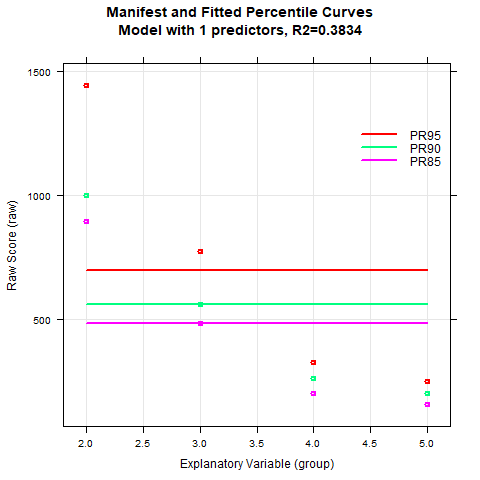The percentile curves can nevertheless be modelled with sufficient quality, at least for the slower decoding times. The floor effect (i.e., the minimum presentation time of 150 ms) is also captured correctly. There are various reasons for the worse model fit as compared to the previous examples. One of these causes, as already mentioned, lies in the extreme skewness and the great floor effect that shows up above grade three. The fact that R2 does not exceed .94 in the finally selected model is also due to the fact that the subtest reliability of rtt =.78 is generally rather low. Overall, the lower boundary for R2 has to be reduced to avoid model overfit.

# Model with four predictors

model.speed <- cnorm(raw=elfe2\$time, group=elfe2\$group, terms=4)

-------------
Output:
User specified solution: 4
Final regression model: raw ~ L4 + L1A3 + L2A2 + L3A1

Beta weights are accessible via 'model\$coefficients':

 (Intercept) L4 L1A3 L2A2 L3A1 1.407712e+02 2.533804e-04 -4.252275e-01 1.068003e-01 -8.940068e-03

Regression formula:
 "raw ~ 140.7712 + (0.00025338036*L4) + (-0.42522753*L1A3) + (0.1068003*L2A2) + (-0.0089400677*L3A1)"

Another way to improve R2 when modeling reaction or processing times is to subject the reaction times to a log transformation beforehand in order to reduce the skewness of the data. In fact, we have carried out such a transformation in the finally published reading comprehension test. Additionally, the subtest was recommended as optional and only to identify very poorly performing children. We have nevertheless used the untransformed processing times in this example to point out possible limits of the modeling procedure in this tutorial.

### Final Conclusion

Modeling large data sets is sometimes reminiscent of the work of sculptors. You take away a little here and add a little there to get a visually satisfying result in the end. In many cases there is no clear right or wrong. Even the best modeling procedure cannot make a good test from an largely unrepresentative or undersized sample or from an unreliable scale. Unfortunately, the biggest errors usually occur in the extreme (especially in the extremely low) normal scores. This applies not only to our, but to all standardization procedures. However, it is precisely these areas that have the highest relevance in diagnostic practice. For example, a normal score in the low range often is crucial for school careers, participation in educational assistance programs or even therapeutic care. Test standardization therefore places high demands on the responsibility, care and effort of the test designer. We hope that cNORM will help to meet these requirements more easily and thus increase diagnostic quality.Generating Norm Tables Graphical User Interface